Publication date: 04/12/2021

## Statistical Details for Smoothing Models

Smoothing models are defined as follows: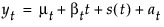where

μt is the time-varying mean term

βt is the time-varying slope term

s(t) is one of the s time-varying seasonal terms

at are the random shocks

Models without a trend have βt = 0 and nonseasonal models have s(t) = 0. The estimators for these time-varying terms are defined as follows:

Lt is a smoothed level that estimates μt

Tt is a smoothed trend that estimates βt

St - j for j = 0, 1,..., s - 1 are the estimates of the s(t)

Each smoothing model defines a set of recursive smoothing equations that describe the evolution of these estimators. The smoothing equations are written in terms of model parameters called smoothing weights:

α is the level smoothing weight

γ is the trend smoothing weight

ϕ is the trend damping weight

δ is the seasonal smoothing weight

While these parameters enter each model in a different way (or not at all), they have the common property that larger weights give more influence to recent data while smaller weights give less influence to recent data.

### Simple Exponential Smoothing

The model for simple exponential smoothing is yt = μt + αt.

The smoothing equation, Lt = αyt + (1 – α)Lt-1, is defined in terms of a single smoothing weight α. This model is equivalent to an ARIMA(0, 1, 1) model where the following is true: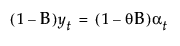where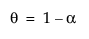The moving average form of the model is defined as follows: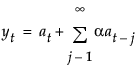### Double (Brown) Exponential Smoothing

The model for double exponential smoothing is yt = μt + β1t + at.

The smoothing equations, defined in terms of a single smoothing weight α, are defined as follows: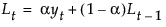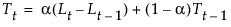This model is equivalent to an ARIMA(0, 1, 1)(0, 1, 1)1 model where the following is true: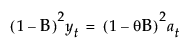where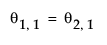withThe moving average form of the model is defined as follows: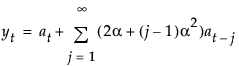### Linear (Holt) Exponential Smoothing

The model for linear exponential smoothing is yt = μt + βtt + at.

The smoothing equations, in terms of smoothing weights α and γ, are defined as follows: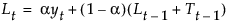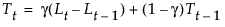This model is equivalent to an ARIMA(0, 2, 2) model where the following is true: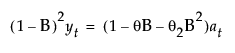with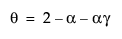and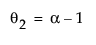The moving average form of the model is defined as follows: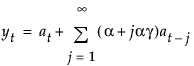### Damped-Trend Linear Exponential Smoothing

The model for damped-trend linear exponential smoothing is yt = μt + βtt + at.

The smoothing equations, in terms of smoothing weights α, γ, and ϕ, are defined as follows: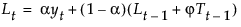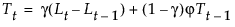This model is equivalent to an ARIMA(1, 1, 2) model where the following is true: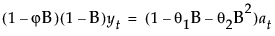where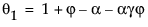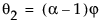The moving average form of the model is defined as follows: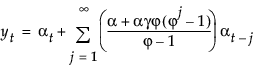### Seasonal Exponential Smoothing

The model for seasonal exponential smoothing is yt = μt + s(t) + at.

The smoothing equations in terms of smoothing weights α and δ are defined as follows: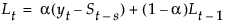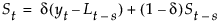This model is equivalent to a seasonal ARIMA(0, 1, s+1)(0, 1, 0)s model: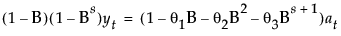where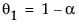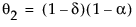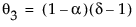The moving average form of the model is defined as follows: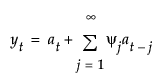where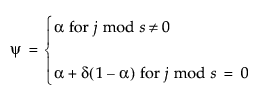### Winters Method (Additive)

The model for the additive version of the Winters method is yt = μt + βtt + s(t) + at.

The smoothing equations in terms of weights α, γ, and δ are defined as follows: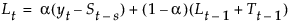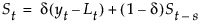This model is equivalent to a seasonal ARIMA(0, 1, s+1)(0, 1, 0)s model defined as follows: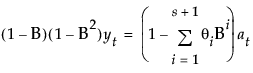The moving average form of the model is defined as follows: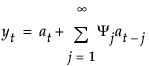where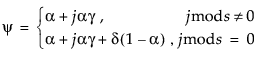Want more information? Have questions? Get answers in the JMP User Community (community.jmp.com).